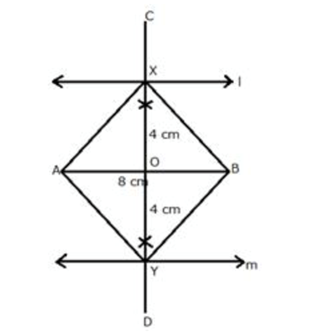Saturday, 23 September 2023, 4:23 PM
Glossary: Ivy Online Glossary
C

D

F

L

#### locus of a point

A straight-line AB is 8 cm long. Draw and describe the locus of a point which is: always 4 cm from the line AB

SolutionStep 1:

Draw a line segment AB of length 8 cm.

Step 2:

Draw two parallel lines l and m to AB at a distance of 4 cm.

Step 3:

Draw the perpendicular bisector of AB which intersects the parallel lines l and m, at X and Y respectively then, X and Y are the required points.

Step 4:

Join AX, AY, BX and BY.

The figure AXBY is a square as its diagonals are equal and intersect at 90o.

M

P

Q